# Recursive model theory

(diff) ← Older revision | Latest revision (diff) | Newer revision → (diff)

recursively presented model theory

A branch of mathematics that is on the border-line between model theory, algebra and the theory of recursive functions (cf. Recursive function), and related to the study of questions of effectiveness in models and algebras.

A.I. Mal'tsev's article Constructive algebras  was the first overviewing work on recursively presented models. In it the basic concepts were developed and systematized, and the road to further development of this theory was indicated. A major role in the erection and development of this branch of mathematics was played by Yu.L. Ershov and his students, who solved a number of fundamental problems, worked out new concepts and determined new directions of research in recursive model theory (cf. ).

The basic notions and results in recursive model theory are formulated below. All considerations are, usually, in a fixed signature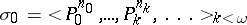such that the function: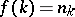is recursive. When considering algebraic systems, function symbols may occur in the signature. The signature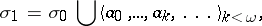which is obtained from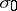by adjoining a countable number of individual constants, is also used. It is always understood that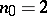and that the predicate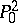is defined on any model as equality. Let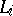,, be the collection of all formulas of restricted predicate calculus with equality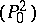of signatures,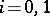, and letbe a fixed Gödel enumeration (cf. ) of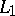(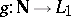). A subsetis called decidable if the setis recursive (cf. Recursive set theory). An enumerated model (of signature) is a pair, where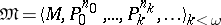is a model of signatureandis a enumeration of the underlying setof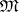. An enumerated modelis called a recursively presented model if the setis recursive.

For each enumerated modelone can construct some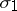-saturation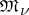of, i.e. a model of signature, with underlying set that of, with predicates fromincoinciding with the corresponding predicates of, and with constants defined by: As the element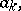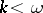, one takes. Let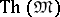be the elementary theory of, i.e. the set of closed formulas of signaturethat are true on. Let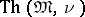be the elementary theory of the enumerated model. An enumerated model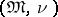is called strongly recursively presented or decidable ifis a decidable theory. From the definition it is immediately clear that a strongly recursively presented model is recursively presented.

One of the basic problems in recursive model theory is the existence of recursively presented models with various elementary properties, i.e. with properties describable in the language of restricted predicate calculus. A number of interesting and important theorems have been obtained (1990) in this direction. The following yields the existence of a large class of strongly recursively presented models: If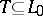is a decidable theory, then there is a sequence of strongly recursively presented models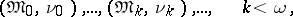such that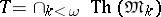and the set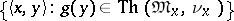is recursive. It has been noticed that there are formulas having no recursively presented models. The following two theorems give some sufficient conditions for the existence of recursively presented models for a theory with a recursively-enumerable set of axioms.

Ifis a recursively-enumerable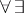-theory having a modelwith a recursively-enumerable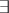-theory, thenhas a recursively presented model.

A theoryof finite signature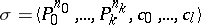is called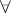-finite if the universal theory of any extension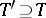(of the same signature) is finitely axiomatizable (by universal statements). A theoryis called strongly-finite if for any finite set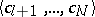of individual constants the theory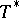is-finite. Hereis defined to bebut now in the language of the signature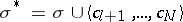.

If a theoryis strongly-finite, and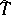is a recursively-enumerable extension of, thenhas a recursively presented model.

Another circle of problems is related to the existence, for a given model, of enumerationssuch thatbecomes a (strongly) recursively presented model. Models for which such an enumeration exists are called (strongly) recursively presentable, while the corresponding enumeration is called a (strong) recursive presentation. For the solution of a number of problems related to the recursive presentability of models, Ershov's kernel theorem turns out to be useful. Its application to concrete algebraic systems allows one to solve a number of natural problems. In particular, it has been established that: 1) any recursively presented locally nilpotent torsion-free group has a recursively presented completion; and 2) ifis a recursively presented field and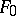is an algebraic extension of, thenextends to a recursive presentation ofif and only if the family of finite sets of polynomials overin a countable number of variables, with roots in, is recursively enumerable.

A large class of recursively presented models is given by the following theorem: Any countable model of an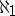-categorical (cf. Categoricity in cardinality) decidable theory is strongly recursively presentable. The problem of the (strong) recursive presentability of special models of complete theories, in particular of simple and universal models, is of interest. Sufficient and necessary conditions for the (strong) recursive presentability of simple (and countable saturated) models of a complete theory have been found. Examples of complete theories with non-recursively presentable simple and universal models have been constructed. It has been proved that a simple model of a complete decidable theory that has a strongly recursively presentable universal model or a finite number of pairwise non-isomorphic countable models, is always strongly recursively presentable.

The question of the number of inequivalent recursive presentations for a given model has been investigated. Two recursive presentationsandof a modelare called (recursively) equivalent if there exists an isomorphism(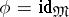) and a recursive functionsuch that. A model is called self-stable (recursively stable) if any two recursive presentations of it are (recursively) equivalent.

For a large class of algebraic systems it has been proved that there exists either only one (up to equivalence) or a countable number of inequivalent recursive presentations , . For the theories of fields, Boolean algebras, torsion-free Abelian groups, and some other classes of algebraic systems the question of the number of inequivalent recursive presentations has been solved completely , and the self-stable models have been described . It has also been demonstrated that questions of self-stability are related to the study of computability of classes of recursively presented models.

How to Cite This Entry:
Recursive model theory. Encyclopedia of Mathematics. URL: http://encyclopediaofmath.org/index.php?title=Recursive_model_theory&oldid=12215
This article was adapted from an original article by S.S. Goncharov (originator), which appeared in Encyclopedia of Mathematics - ISBN 1402006098. See original article